Illustrated quantile calculations from distributions

qdist(
dist = "norm",
p,
plot = TRUE,
verbose = FALSE,
invisible = FALSE,
resolution = 500L,
digits = 3L,
xlim,
ylim,
return = c("values", "plot"),
refinements = list(),
...
)

xqgamma(
p,
shape,
rate = 1,
scale = 1/rate,
lower.tail = TRUE,
log.p = FALSE,
...
)

xqt(p, df, ncp, lower.tail = TRUE, log.p = FALSE, ...)

xqchisq(p, df, ncp = 0, lower.tail = TRUE, log.p = FALSE, ...)

xqf(p, df1, df2, lower.tail = TRUE, log.p = FALSE, ...)

xqbinom(p, size, prob, lower.tail = TRUE, log.p = FALSE, ...)

xqpois(p, lambda, lower.tail = TRUE, log.p = FALSE, ...)

xqgeom(p, prob, lower.tail = TRUE, log.p = FALSE, ...)

xqnbinom(p, size, prob, mu, lower.tail = TRUE, log.p = FALSE, ...)

xqbeta(p, shape1, shape2, ncp = 0, lower.tail = TRUE, log.p = FALSE, ...)

## Arguments

dist

a character description of a distribution, for example "norm", "t", or "chisq"

p

a vector of probabilities

plot

a logical indicating whether a plot should be created

verbose

a logical

invisible

a logical

resolution

number of points used for detecting discreteness and generating plots. The default value of 5000 should work well except for discrete distributions that have many distinct values, especially if these values are not evenly spaced.

digits

the number of digits desired

xlim

x limits. By default, these are chosen to show the central 99.8\ of the distribution.

ylim

y limits

return

If "plot", return a plot. If "values", return a vector of numerical values.

refinements

A list of refinements to the plot. See ggformula::gf_refine().

...

additional arguments, including parameters of the distribution and additional options for the plot. To help with name collisions (eg size for binomial distributions and shape for gamma distributions), argument names beginning plot_ will be renamed to remove plot_ and passed only to the plot. The unprefixed version will used as a parameter for the the distribution.

shape

shape and scale parameters. Must be positive, scale strictly.

rate

an alternative way to specify the scale.

scale

shape and scale parameters. Must be positive, scale strictly.

lower.tail

logical; if TRUE (default), probabilities are $$P[X \le x]$$, otherwise, $$P[X > x]$$.

log.p

logical; if TRUE, probabilities/densities $$p$$ are returned as $$log(p)$$.

df

degrees of freedom ($$> 0$$, maybe non-integer). df = Inf is allowed.

ncp

non-centrality parameter $$\delta$$; currently except for rt(), only for abs(ncp) <= 37.62. If omitted, use the central t distribution.

df1

degrees of freedom. Inf is allowed.

df2

degrees of freedom. Inf is allowed.

size

number of trials (zero or more).

prob

probability of success on each trial.

lambda

vector of (non-negative) means.

mu

alternative parametrization via mean: see ‘Details’.

shape1

non-negative parameters of the Beta distribution.

shape2

non-negative parameters of the Beta distribution.

## Value

a vector of quantiles; a plot is printed as a side effect

## Details

The most general function is qdist which can work with any distribution for which a q-function exists. As a convenience, wrappers are provided for several common distributions.

## Examples

qdist("norm", seq(.1, .9, by = 0.10),
title = "Deciles of a normal distribution", show.legend = FALSE,
pattern = "rings")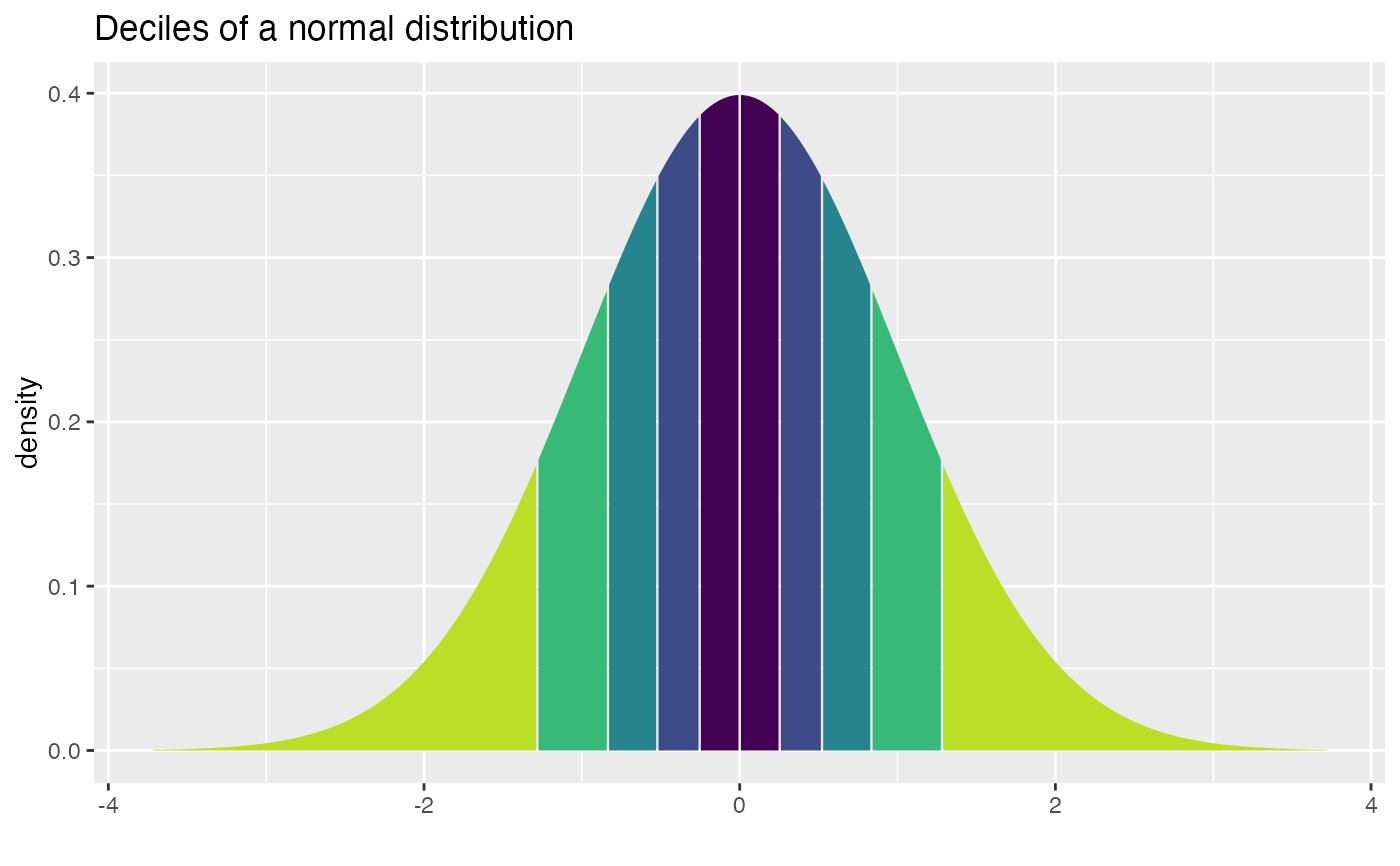#>  -1.2815516 -0.8416212 -0.5244005 -0.2533471  0.0000000  0.2533471  0.5244005
#>   0.8416212  1.2815516
xqnorm(seq(.2, .8, by = 0.20), mean = 100, sd = 10)
#>
#> If X ~ N(100, 10), then
#> 	P(X <=  91.58379) = 0.2	P(X <=  97.46653) = 0.4	P(X <= 102.53347) = 0.6	P(X <= 108.41621) = 0.8
#> 	P(X >   91.58379) = 0.8	P(X >   97.46653) = 0.6	P(X >  102.53347) = 0.4	P(X >  108.41621) = 0.2
#>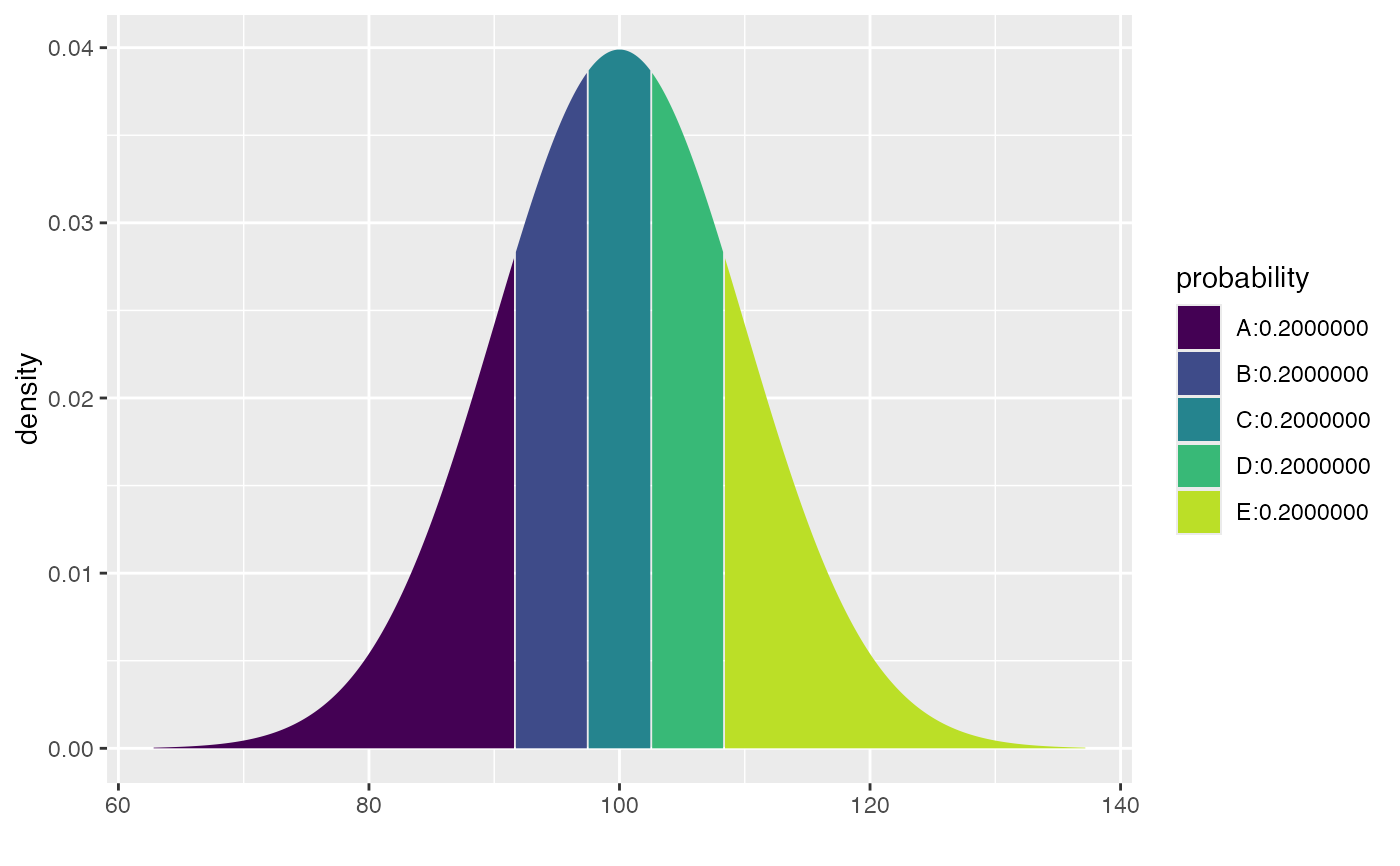#>   91.58379  97.46653 102.53347 108.41621
qdist("unif", .5)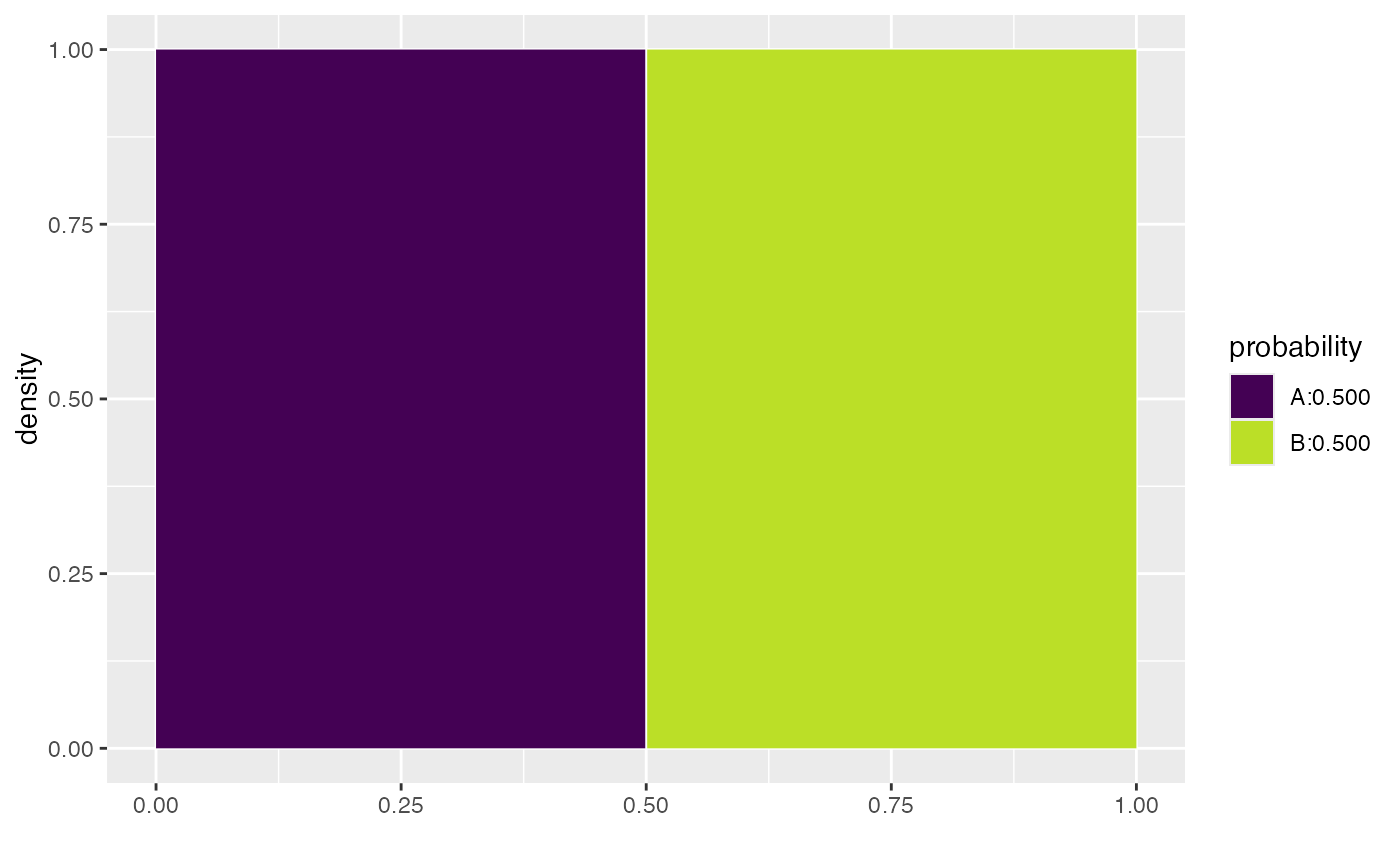#>  0.5
xqgamma(.5, shape = 3, scale = 4)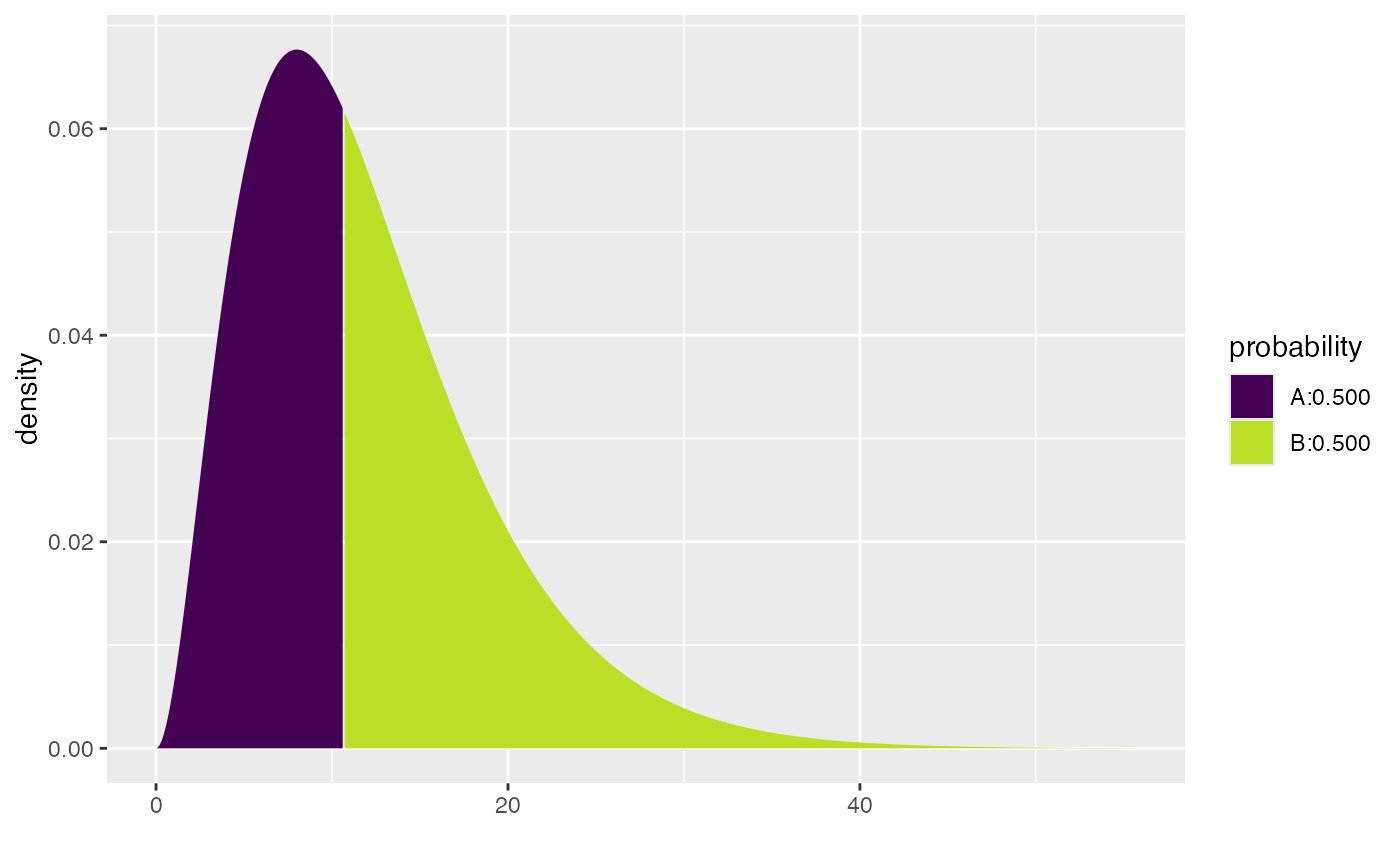#>  10.69624
xqgamma(.5, shape = 3, scale = 4, color = "black")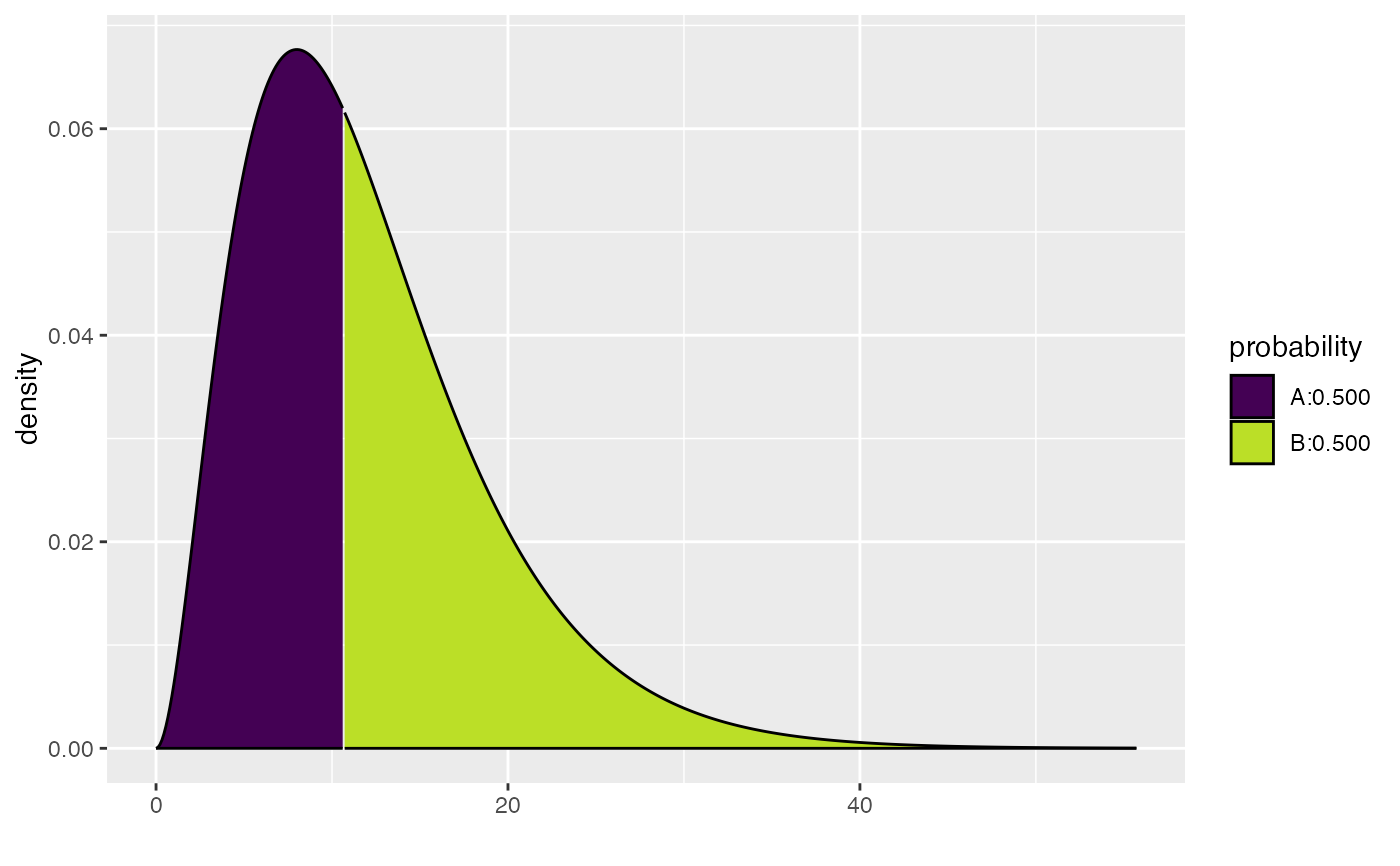#>  10.69624
xqbeta(.5, shape1 = .9, shape2 = 1.4, dlwd = 1)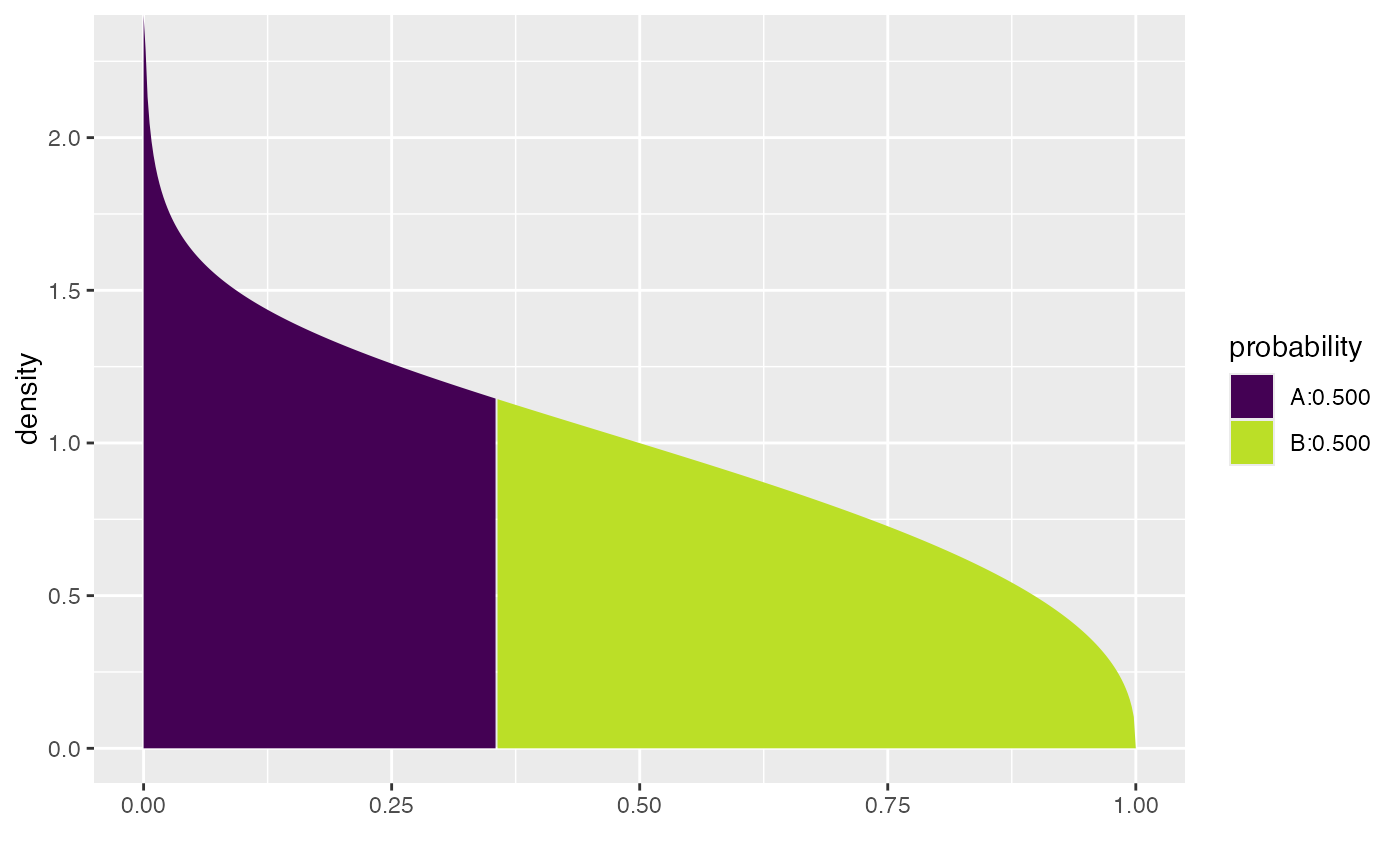#>  0.3557401
xqchisq(c(.25,.5,.75), df = 3)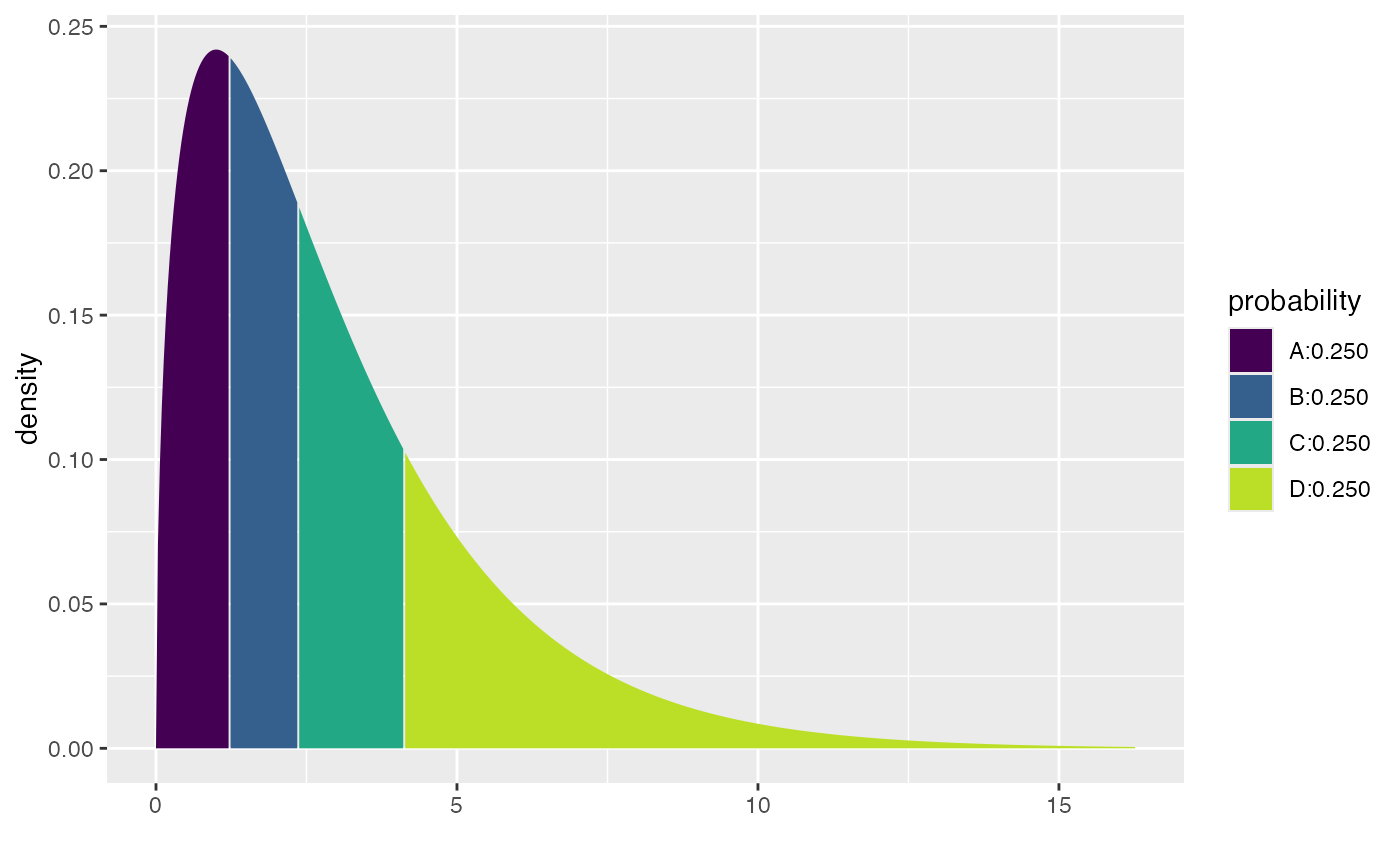#>  1.212533 2.365974 4.108345
xcbinom(c(0.80, 0.90), size = 1000, prob = 0.40)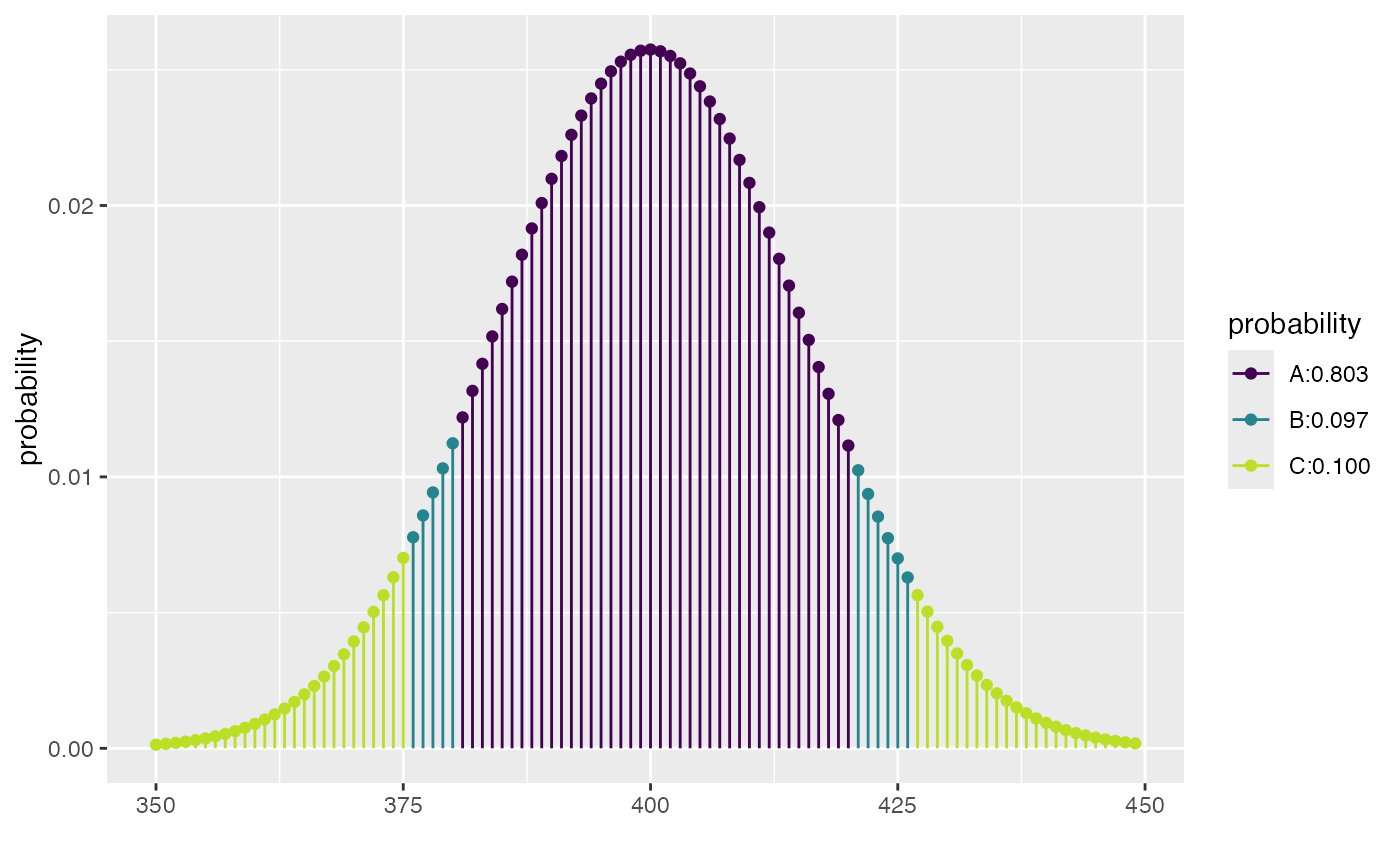#>  375 380 420 426
# displayed as if continuous
xcbinom(c(0.80, 0.90), size = 5000, prob = 0.40)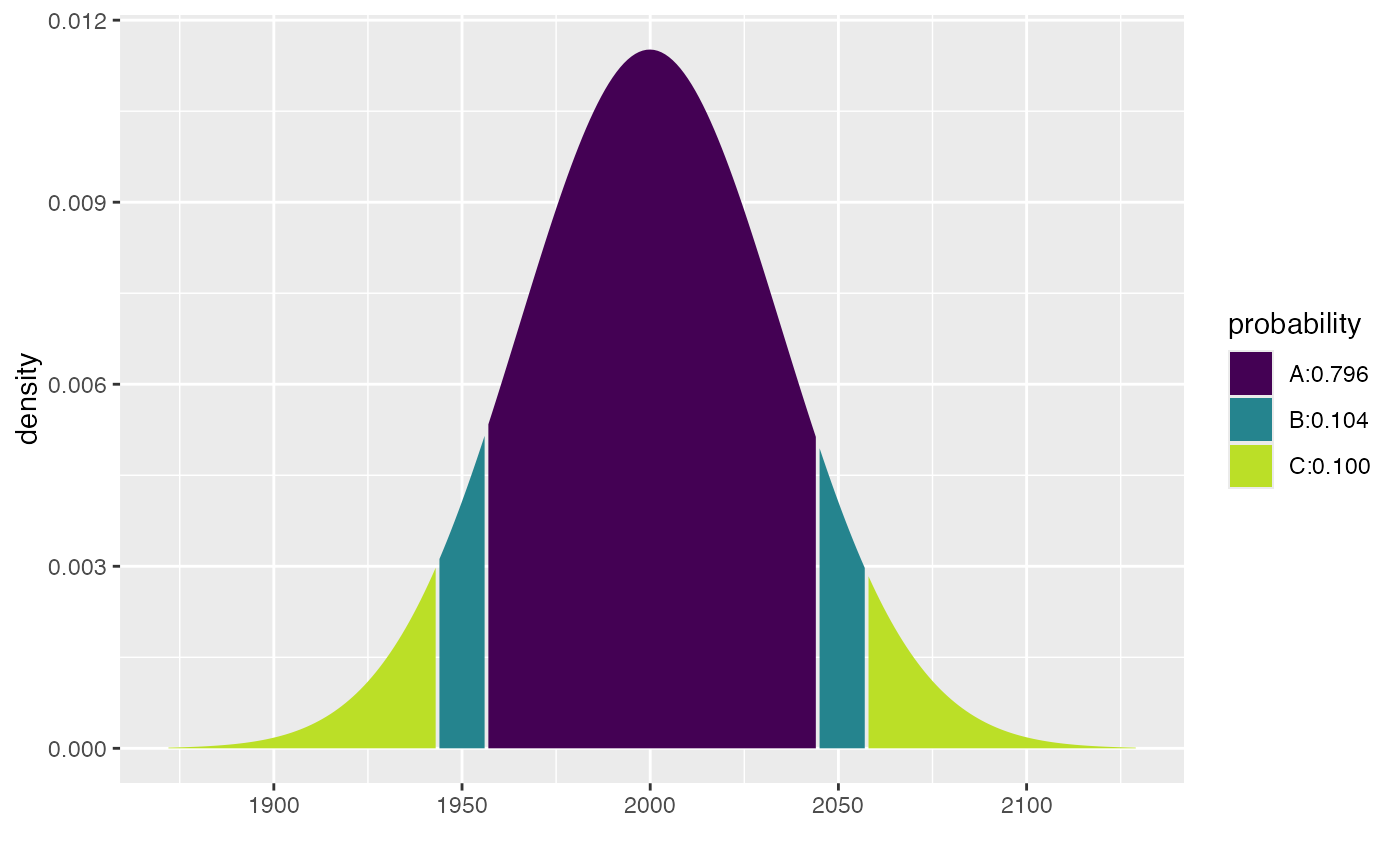#>  1943 1956 2044 2057
xpbinom(c(480, 500, 520), size = 1000, prob = 0.48)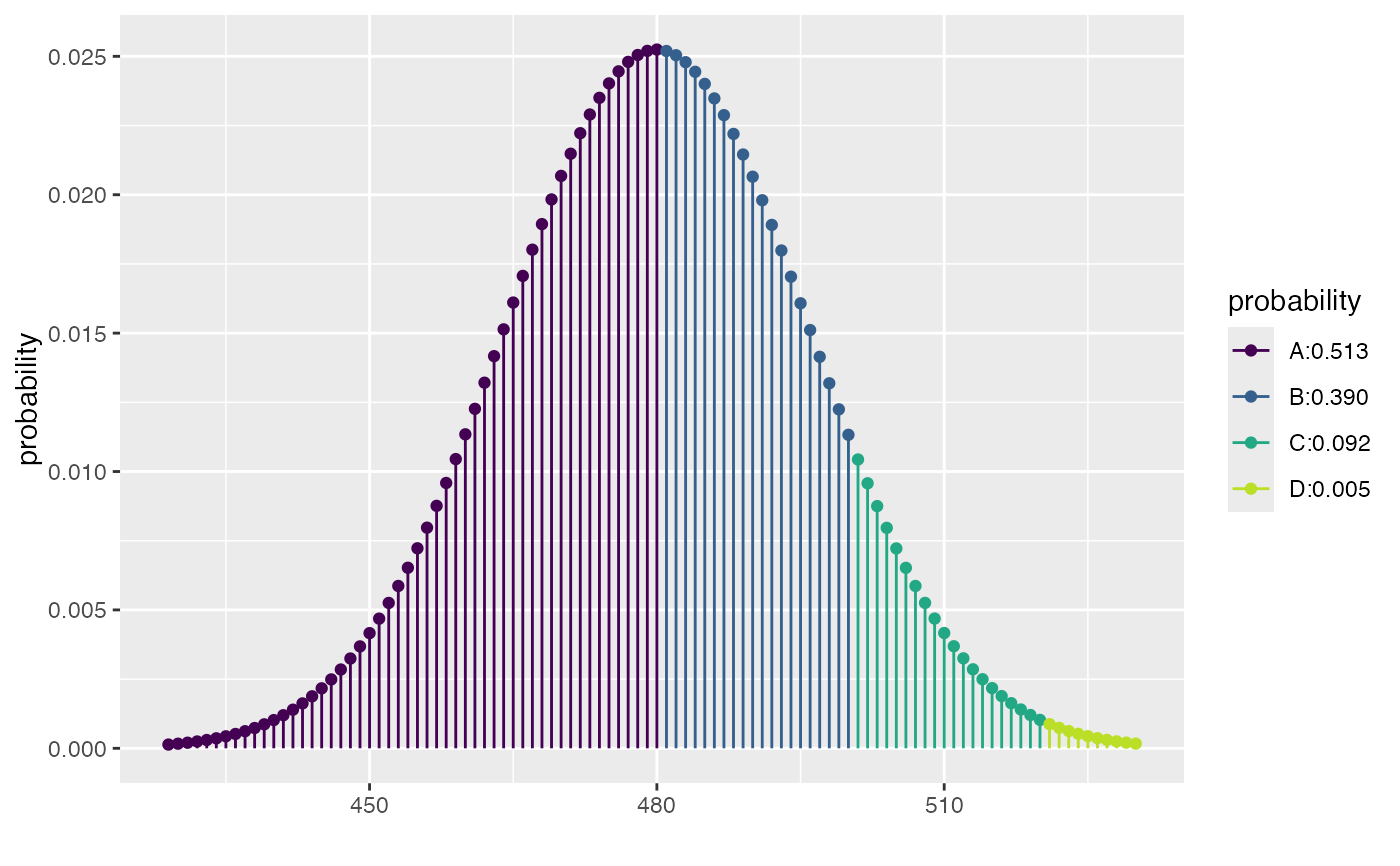#>  0.5127908 0.9027460 0.9948015
xpbinom(c(40, 60), size = 100, prob = 0.5)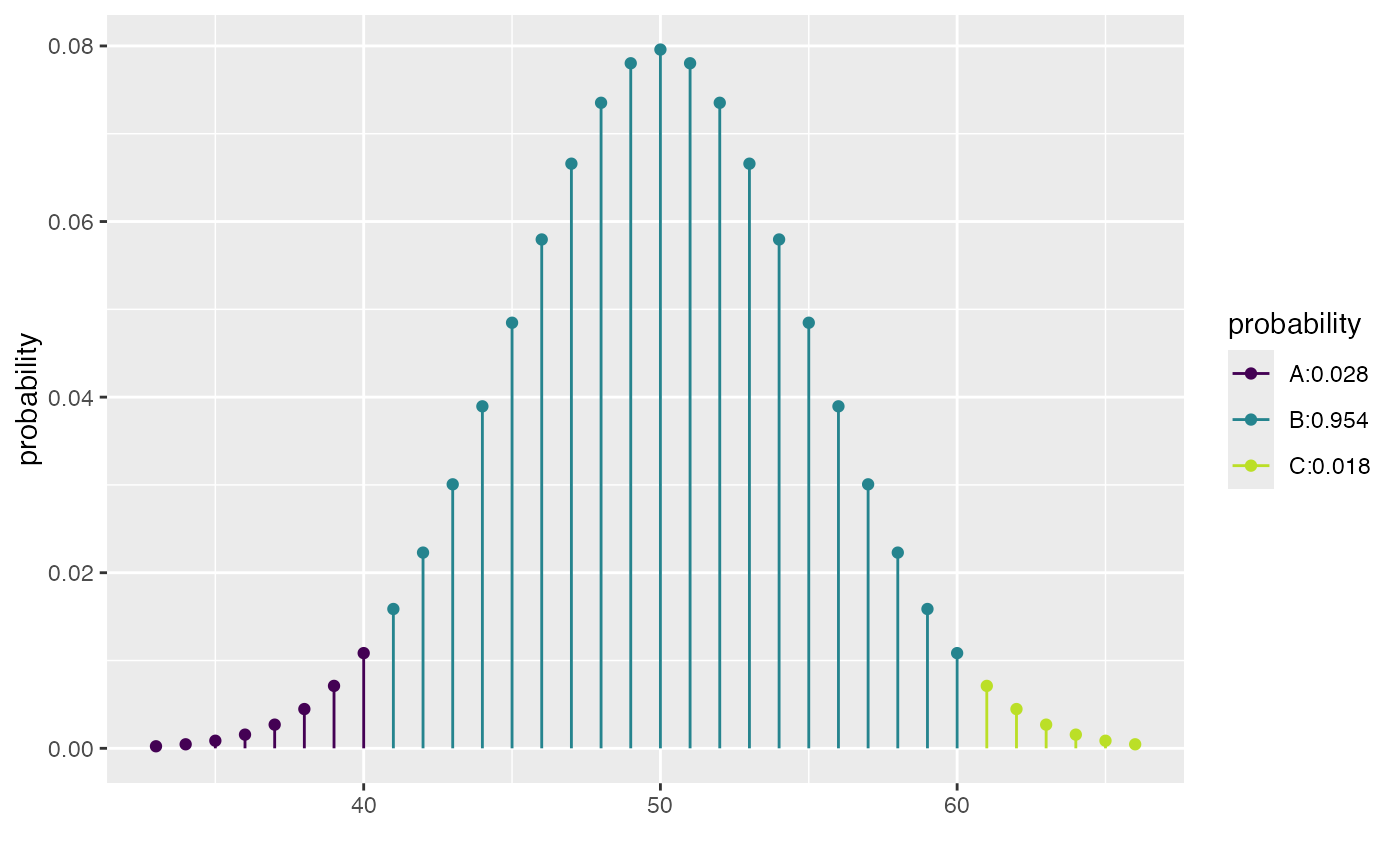#>  0.02844397 0.98239990
xqpois(c(0.25, 0.5, 0.75), lambda = 12)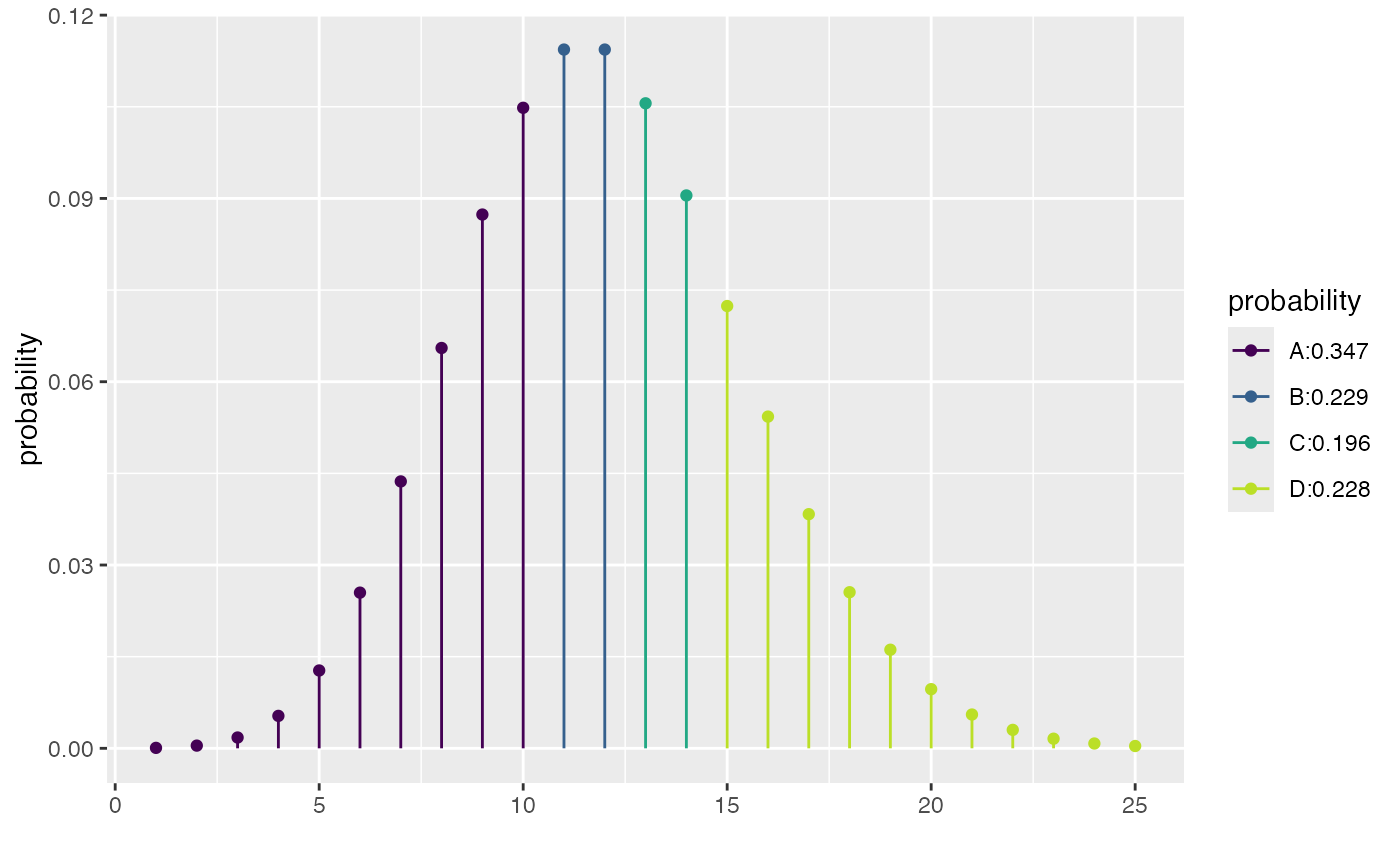#>  10 12 14
xcpois(0.50, lambda = 12)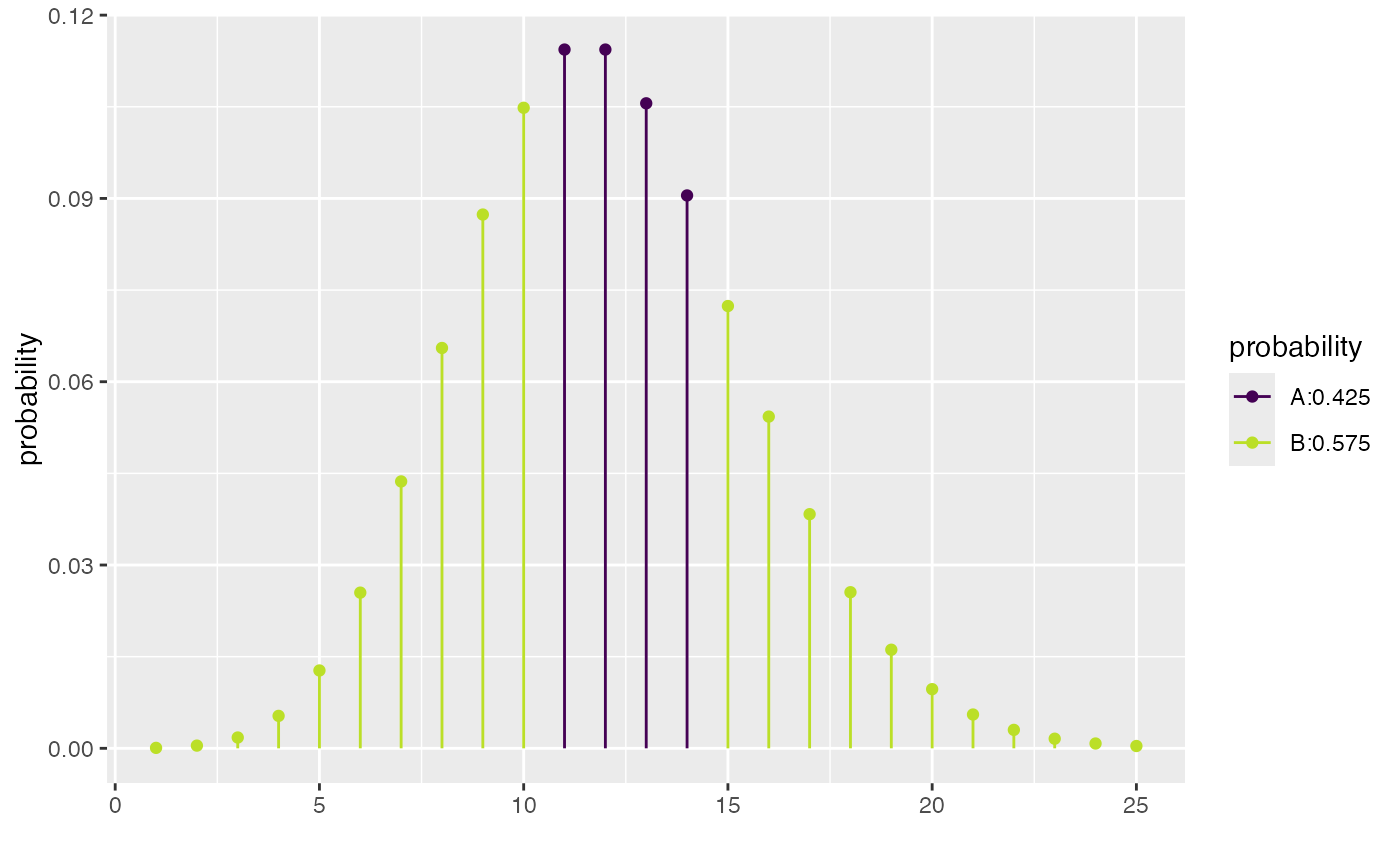#>  10 14
xcpois(0.50, lambda = 12, refinements = list(scale_color_brewer(type = "qual", palette = 5)))
#> Scale for 'colour' is already present. Adding another scale for 'colour',
#> which will replace the existing scale.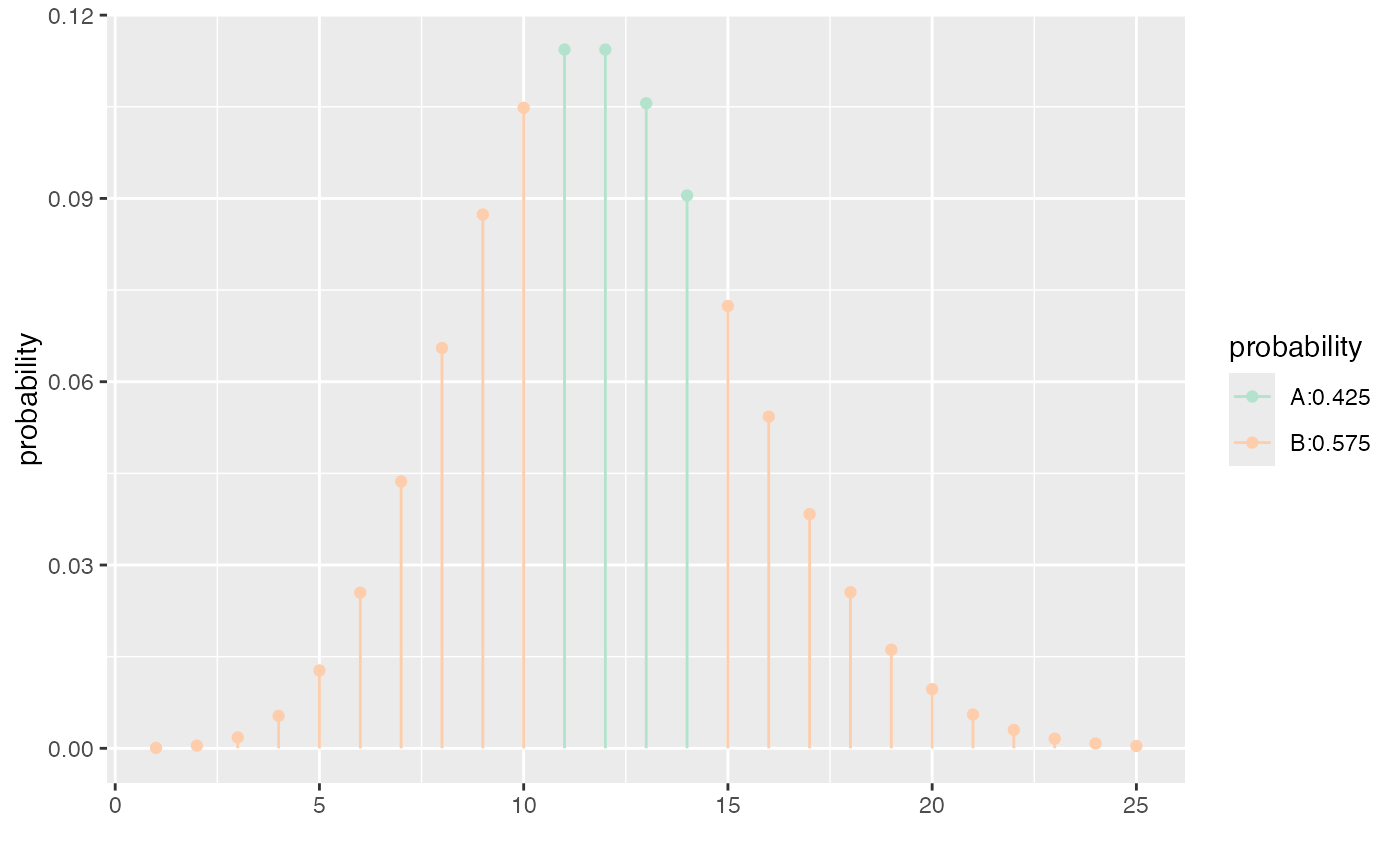#>  10 14